# General linear group

(diff) ← Older revision | Latest revision (diff) | Newer revision → (diff)

The group of allinvertible matrices over an associative ring (cf. Associative rings and algebras)with a unit; the usual symbols areor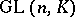. The general linear groupcan also be defined as the automorphism group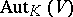of the free right-modulewithgenerators.

In research on the groupits normal structure is of considerable interest. The centre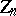of the groupconsists of scalar matrices with entries from the centre of the ring (cf. Centre of a ring). Whenis commutative one defines the special linear group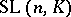, which consists of matrices with determinant 1. Whenis a field, the commutator subgroup of the groupcoincides with(apart from the case,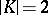), and any normal subgroup ofis either contained inor contains. In particular, the projective special linear groupis a simple group (apart from the cases,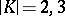).

Ifis a skew-field and, any normal subgroup ofis either contained inor contains the commutator subgroup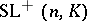ofgenerated by transvections (cf. Transvection), and the quotient group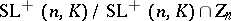is simple. Also, there exists a natural isomorphismwhere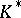is the multiplicative group of the skew-field. Ifis finite-dimensional over its centre, then the role ofis played by the group of all matrices fromwith reduced norm 1. The groupsanddo not always coincide, although this is so ifis a global field (see Kneser–Tits hypothesis).

The study of the normal structure of general linear groups over a ringis associated with algebraic-theory. The groupover a general ringmay contain numerous normal subgroups. For example, ifis a commutative ring without zero divisors and with a finite number of generators, thenis a residually-finite group, i.e. for each elementthere exists a normal subgroup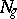of finite index not containing. In the case, the description of the normal subgroups of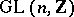is in fact equivalent to the congruence problem for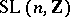, since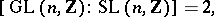and any non-scalar normal subgroup of the groupfor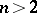is a congruence subgroup.

There is a deep analogy between the structure of general linear groups and that of other classical groups. This analogy extends also to simple algebraic groups and Lie groups.

How to Cite This Entry:
General linear group. Encyclopedia of Mathematics. URL: http://encyclopediaofmath.org/index.php?title=General_linear_group&oldid=13698
This article was adapted from an original article by V.P. Platonov (originator), which appeared in Encyclopedia of Mathematics - ISBN 1402006098. See original article